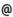## "Babes-Bolyai" University of Cluj-Napoca Faculty of Mathematics and Computer Science

 Parallel calculus
 Code Semes-ter Hours: C+S+L Credits Type Section MC012 8 2+2+0 10 optional Informatică
 Teaching Staff in Charge
 Assoc.Prof. CHIOREAN Ioana Rodica, Ph.D., ioanacs.ubbcluj.ro
 Aims Introduction of the basic notions of parallel calculus, in order to get aquainted with the writing, omplementing and processing the programs with more than one processor.
 Content 1. The necessity of supercomputers. Motivation "for" and "against" parallel calculus. 2. Parallel programming models (pipeline, processors array, shared memory, passing messages). Taxonomies for parallel computers (Flynn, Hockney, Schwartz, Handler). Measures of performance for parallel algorithms. 3. Processors networks. 4. Double-recursive technique and its application at reccurence evaluation. Odd-even reduction technique. Slicing and crinkling techniques. 5. Parallel Data Transfromation (PDT). 6. Parallel matrix multiplication. Parallel evaluation of arithmetic expressions. 7. Parallel bit algorithms. 8. Parallel sorting algorithms ( bitonic sort, bubble-sort, quick-sort, tree-sort, odd-even transpozition sort). 9. Parallel algorithms in computational biology (DNA and RNA sequences comparison, etc.) 10.Programming in PVM (Parallel Virtual Machine)
 References 1. CHIOREAN, I., Calcul paralel. Fundamente. Ed. Microinformatica, 1995 2. COMAN,GH., CHIOREAN,I., Metode numerice si calcul paralel, lito Univ.Babes-Bolyai, 2001 3. CHAUDURI, P., Parallel Algorithms, Design and Analysis, Advanced in Computer Science, Prentice Hall, 1992 4. HOCKNEY, R.W., JESSOPE, C.R., Calculatoare paralele, 1987 5. PETCU, D., Parallel numerical algorithms, (vol.1 si 2), Litografia Universitatii din Timisoara, 1996
 Assessment Coloquium.